# Test Prep Plan - Take a practice test

Take this practice test to check your existing knowledge of the course material. We'll review your answers and create a Test Prep Plan for you based on your results.
How Test Prep Plans work
1
2Based on your results, we'll create a customized Test Prep Plan just for you!
3Study smarter
Study more effectively: skip concepts you already know and focus on what you still need to learn.

# Graphing Derivatives & L'Hopital's Rule - AP Calculus: Homeschool Curriculum Chapter Exam

Exam Instructions:

Choose your answers to the questions and click 'Next' to see the next set of questions. You can skip questions if you would like and come back to them later with the yellow "Go To First Skipped Question" button. When you have completed the practice exam, a green submit button will appear. Click it to see your results. Good luck!

### Page 1

#### Question 1 1. What is the slope of the tangent to r(s) at s = 0?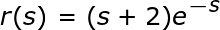#### Question 2 2. Of the three limits shown below, which can be evaluated using L'Hopital's Rule?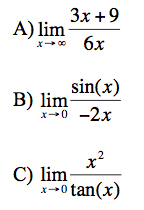#### Question 3 3. Consider the function f(x) below. At x = 2 is this function concave up, concave down, or an inflection point?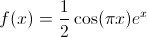#### Question 4 4. Evaluate the limit shown below: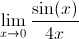#### Question 5 5. What is the slope of a line normal to f(x)?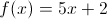### Page 2

#### Question 6 6. Consider the function h(x) below. At x = 1 is this function concave up, concave down, or an inflection point?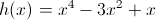#### Question 7 7. Evaluate the limit shown below: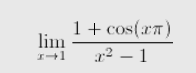#### Question 10 10. Between x=1 and x=3, the following function _____.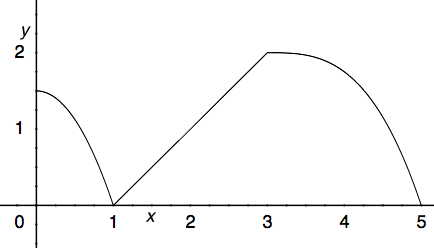### Page 3

#### Question 11 11. Find the following limit: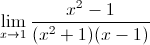#### Question 12 12. Find the following limit: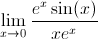#### Question 13 13. Where is the global maximum for the function y=f(x) below between x=0 and x=2?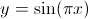#### Question 14 14. What is the equation of the line normal to f(x) at the point x=1?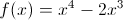#### Question 15 15. If the derivative of a function is plotted below, what might the original function look like?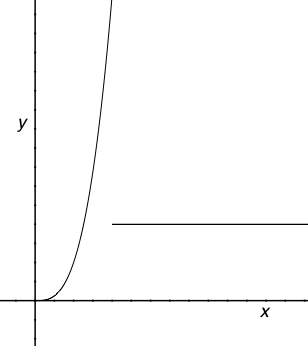### Page 4

#### Question 18 18. What is value of y at the finite local maximum for the function y=f(x) below?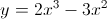#### Question 19 19. What is the slope of the line normal to f(x) at x=1?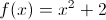### Page 5

#### Question 21 21. Use L'Hopital's rule to evaluate the limit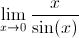#### Question 22 22. Which of the following graphs is the derivative of the graph below?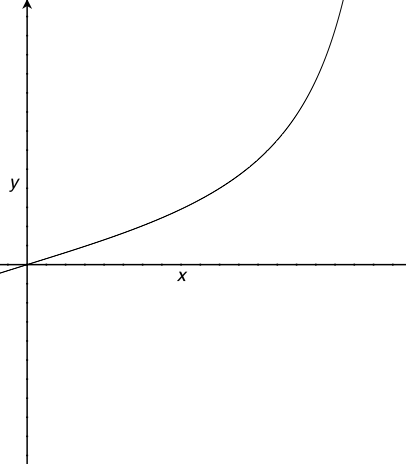#### Question 23 23. The derivative of the following graph is _____ at point D.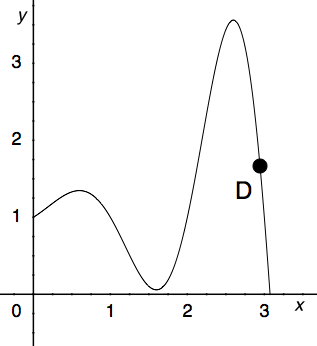#### Question 24 24. Over the region shown, point A is known as _____.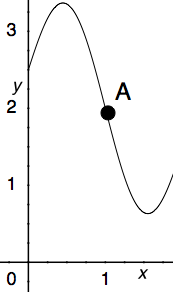#### Question 25 25. Over the region shown, the global minimum is at the point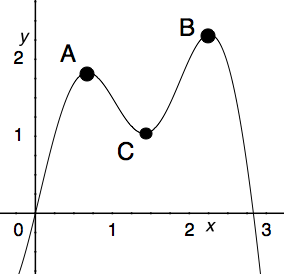### Page 6

#### Question 27 27. Find the following limit: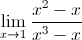#### Question 28 28. Which could be the graph of the derivative of the function below?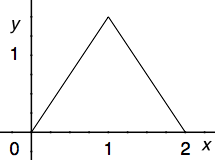#### Question 29 29. Evaluate the limit shown below: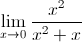#### Question 30 30. Find the following limit: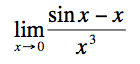#### Graphing Derivatives & L'Hopital's Rule - AP Calculus: Homeschool Curriculum Chapter Exam Instructions

Choose your answers to the questions and click 'Next' to see the next set of questions. You can skip questions if you would like and come back to them later with the yellow "Go To First Skipped Question" button. When you have completed the practice exam, a green submit button will appear. Click it to see your results. Good luck!

Support